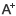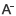# 一年级下册数学第一~三单元训练题日期：2020-11-06     来源：www.zhixueshuo.com    作者：智学网    浏览：457    评论：0

一、口算。

13-9=　　16-7=　　12-3=　　11-8=　　17-9=　　18-9=　　15-8=

16-9=　　14-5=　　15-6=　　11-7=　　14-7=　　12-5=　　13-4=

11-2=　　12-6=　　11-9=　　13-8=　　11-3=　　14-9=　　15-7=

11-4=　　15-5=　　5+6=　　4+9=　　3+8=　　11-5=　　11-6=

12-4=　　12-7=　　12-8=　　12-9=　　13-5=　　13-6=　　13-7=

14-6=　　14-8=　　15-9=　　16-8=　　17-8　　=4+7　　=8+5=

14-9-2=　　12-7+6=　　7+6-8=　　6+3+4=　　11-9+8=　　8+8-7=

二、在（）内填上“>”“<”或“=”。

15-8（）10　　11-3（）11-4　　18-9（）12-2

13-9（）5　　15-10（）14-9　　13-7（）13-6

三、在（）里填上适合的数。
12-（）=6　　4+（）=13　　15-（）=8

7+（）=10　　（）-6=12　　11-（）=7

12+（）=13　　14-（）=8　　5+4=（）-6

12-4=（）-（）=（）-（）=（）-（）=（）-（）

13-7=（）-（）=（）-（）=（）-（）=（）-（）

四、连一连。

8+7　　　　10　　　　13-10

14-4　　　　15　　　　9-7

6+7　　　　3　　　　9+6

7-4　　　　13　　　　5+8

10-8　　　　2　　　　5+5

1、口算题。

13-6=　　12-8=　　13-7=　　5+8=

14-9=　　9+9=　　12+5=　　13-3=

0+10=　　20-5=　　18-6=　　14+6=

11-5=　　17-9=　　6+13=　　15-7=

二、下面的□里分别填几？

5+（）=9　　14-（）=11　　9+（）=13

（）+7=15　　12-（）=6　　4+8=5+（）

三、从8、12、7、15中选三个数写出4个算式
（）+（）=（）
（）+（）=（）
（）-（）=（）
（）-（）=（）

四、在○里填上“>”“<”或“=”。

18-9○17-8　　16-8○5+0　　15-6○18-9

12-5○13-6　　14-6○15-7　　16-7○15-8

五、按需要画一画。

⑴画△比○多5个。

○○○○○○○

______________________________

⑵画□比☆少3个。

☆☆☆☆☆☆☆☆☆☆

____________________________________

一、我会填空。

1、96是由（）个十和（）一组成的。

2、6个十加3个十，和是（）个十。

3、46比40多（），20比54少（）。

4、写出个位是5的数（答案）（答案）（答案）。写出十位是3的数（答案）（答案）。（答案不）

5、先找规律再填数：56、52、48、44、（）、（）、32。

6、读数和写数都从（）起。

7、一个两位数，个位上的数是6，十位上的数比个位上的数多2，这个数是（）。

8、在数位表中，从右侧起第三位是（）位，第二位是（）位。

9、的两位数是（），最小的两位数是（），的两位比最小的两位数多（）。

二、写出下面各数。

二十三写作（）五十四写作（）三十一写作（）

四十八写作（）七十写作（）八十八写作（）

六十三写作（）九十写作（）一百写作（）

三、读出下面的数。

45读作（）56读作（）83读作（）

27读作（）11读作（）34读作（）

19读作（）68读作（）72读作（）

四、把下列各数按需要排列起来。

（1）39，66，60，97，79

（）<（）<（）<（）<（）

（2）45，58，69，56，92

（>（）>（）>（）>（）

五、计算。

20+9-8=　　5+30+2=　　69-9+5=　　84-4+6=
18-9+9=　　80-20+4=　　57-7+10=　　92-2+7=
27+2-6=　　72-70+9=　　17+10-7=　　36-20-4=

0相关评论